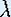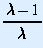Up: Bifurcation

# One-Dimensional Dynamical Systems

## Part 5: Bifurcation

#### Qualitative change of dynamics

The theorem of Hartman and Grobman gives us the relationship between the slope of the graph at a fixed point and whether this fixed point is attracting, repelling, or neutral. For the Logistic map, we have already seen that the fixed point other than 0 is attracting for= 1.5, because the slope is between -1 and 1. However, it is repelling for= 3.1, since the graph of the derivative map no longer has a slope between -1 and 1. Hence, as we increase, the fixed point changes from being attracting to being repelling. This scenario is called a bifurcation.
• Recall that p is a fixed point of f if f(p) = p. Verify that the Logistic family f(x) =x (1 - x) has fixed points x = 0 and x =.

Compute the slope of the graph of the Logistic map at the fixed point 0 in terms of. What is the slope for the other fixed point?

• For what values ofare the fixed points of the Logistic family attracting? Repelling? Neutral? Test your values using the computer.

Up: BifurcationThe Geometry Center Home Page

Written by Hinke Osinga